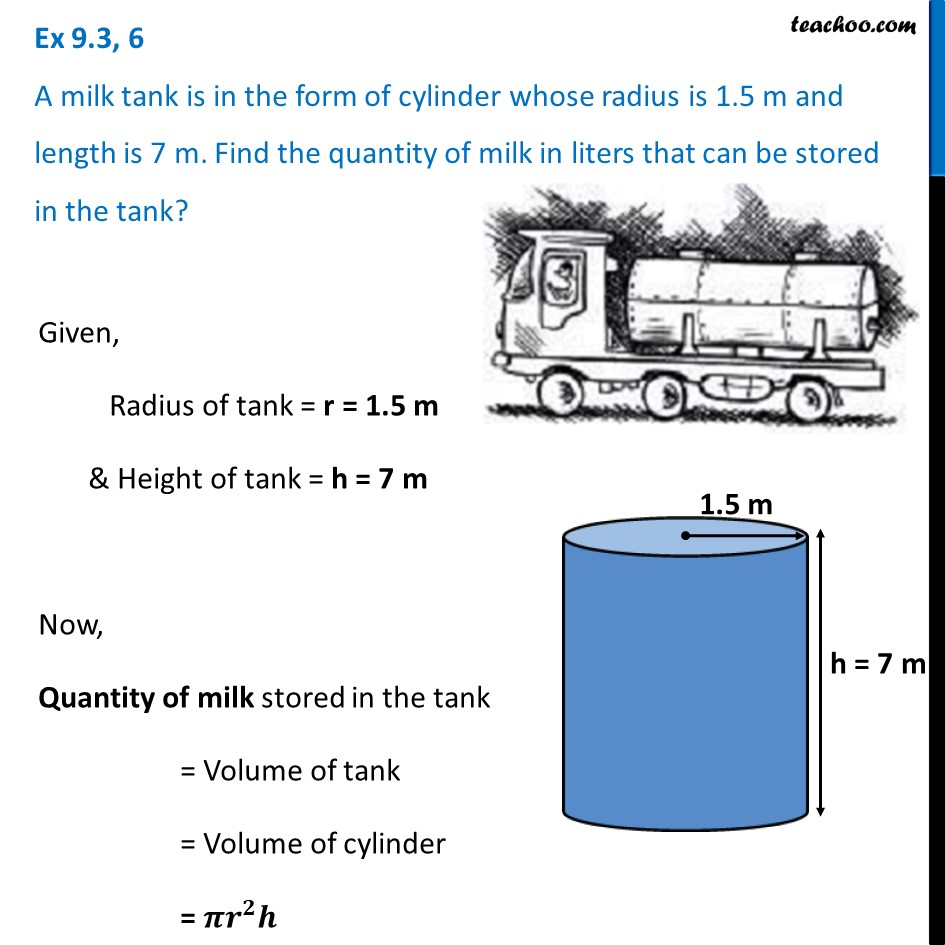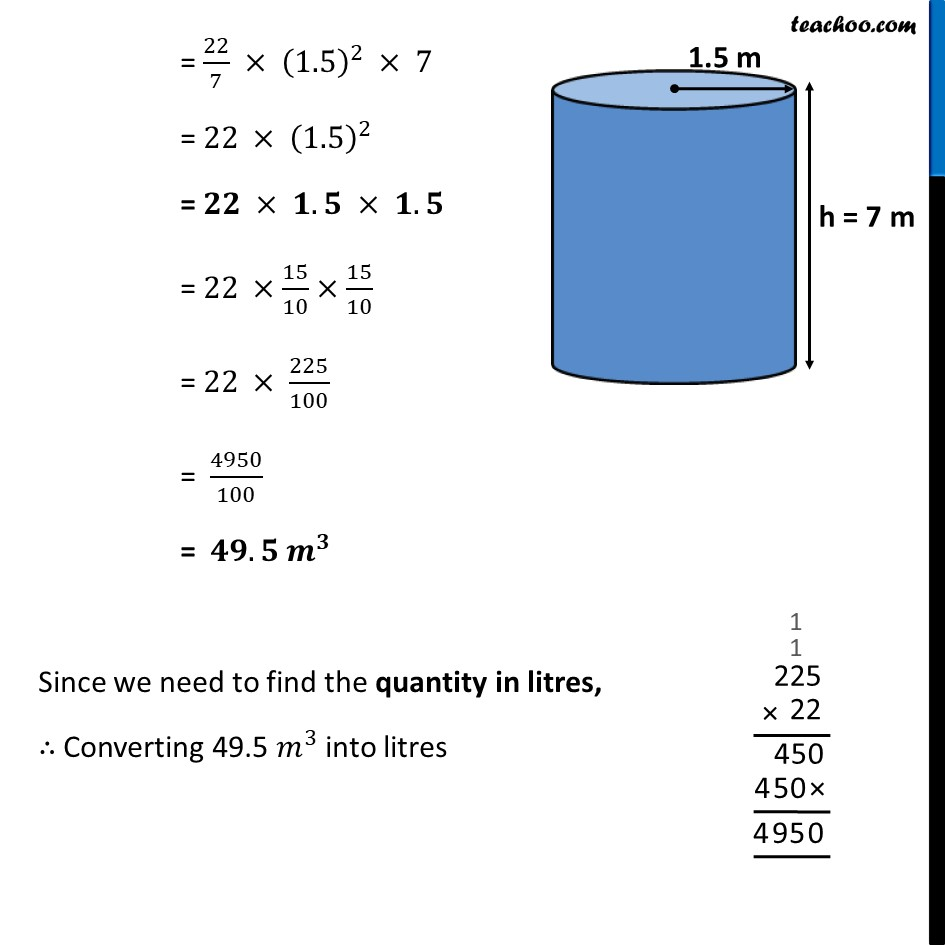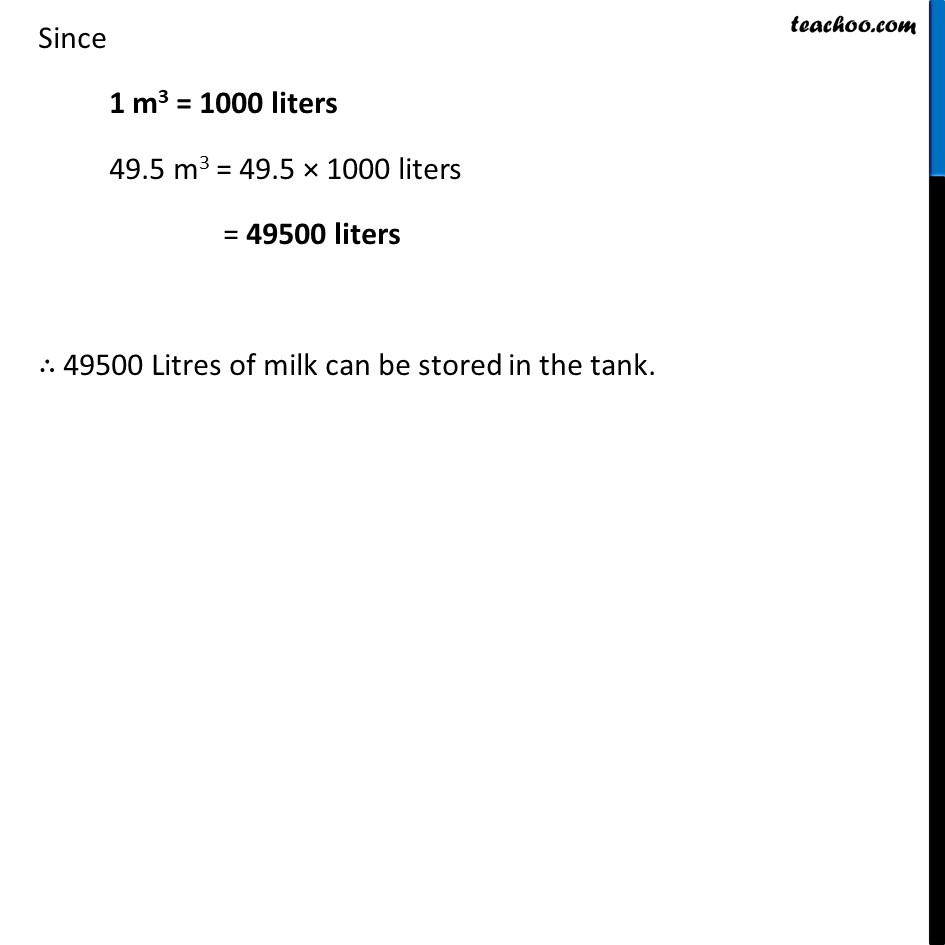Ex 9.3

Chapter 9 Class 8 Mensuration
Serial order wiseLearn in your speed, with individual attention - Teachoo Maths 1-on-1 Class

### Transcript

Ex 9.3, 6 A milk tank is in the form of cylinder whose radius is 1.5 m and length is 7 m. Find the quantity of milk in liters that can be stored in the tank?Given, Radius of tank = r = 1.5 m & Height of tank = h = 7 m Now, Quantity of milk stored in the tank = Volume of tank = Volume of cylinder = 𝝅𝒓^𝟐 𝒉 = 22/7 × (1.5)^2 × 7 = 22 × (1.5)^2 = 𝟐𝟐 × 𝟏.𝟓 × 𝟏.𝟓 = 22 ×15/10×15/10 = 22 × 225/100 = 4950/100 = 𝟒𝟗.𝟓 𝒎^𝟑 Since we need to find the quantity in litres, ∴ Converting 49.5 𝑚^3 into litres Since 1 m3 = 1000 liters 49.5 m3 = 49.5 × 1000 liters = 49500 liters ∴ 49500 Litres of milk can be stored in the tank.